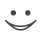Zu dieser Karteikarte gibt es einen kompletten Satz an Karteikarten. Kostenlos!

133
What is the quoted discount rate on a money market instrument?
A.The interest rate earned as a percentage of the final face value of a bond
B.The interest rate earned as a percentage of the initial price of a bond
C.The interest rate earned as a percentage of the average price of a bond
D.The risk-free rate used to calculate the present value of future cash flows from a bond